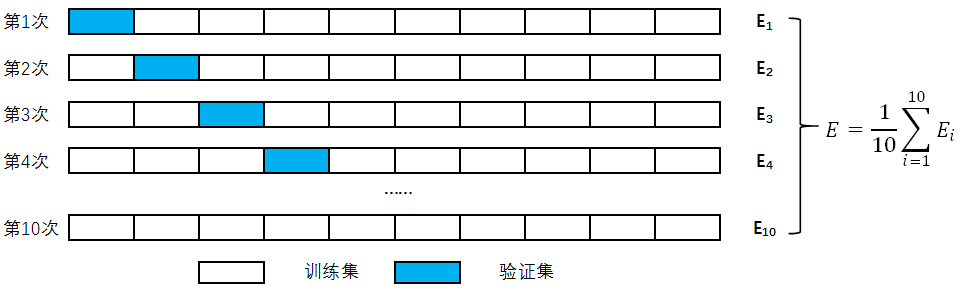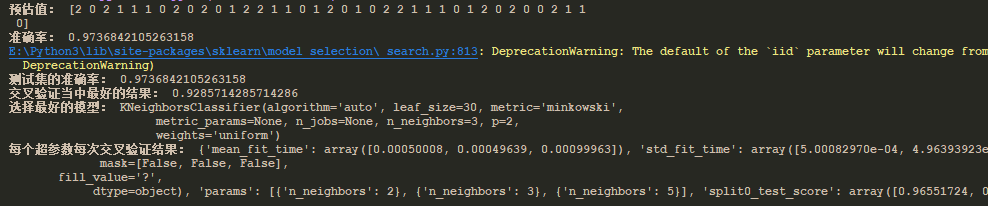# Python之网格搜索与检查验证-5.2

一、网格搜索，在我们不确定超参数的时候，需要通过不断验证超参数，来确定最优的参数值。这个过程就是在不断，搜索最优的参数值，这个过程也就称为网格搜索

二、检查验证，将准备好的训练数据进行平均拆分，分为训练集验证集。训练集和验证集的大小差不多，总体份数通过手动设置。具体过程为：由上图可以得知，训练集和验证集是通过交叉的方式去不断训练，这样的目的就是为了获取，更加优化的参数值。

三、代码演示（这里我们通过K-近邻的算法。来确认参数值）：

# K-近邻算法
def k_near_test():
# 1、原始数据
# print(li.data)
# print(li.DESCR)
# 2、处理数据
data = li.data
target = li.target
x_train, x_test, y_train, y_test = train_test_split(data, target, test_size=0.25)
# 3、特征工程
std = StandardScaler()
x_train = std.fit_transform(x_train, y_train)
x_test = std.transform(x_test)
# 4、算法
knn = KNeighborsClassifier(n_neighbors=2)
knn.fit(x_train, y_train)
# 预估
y_predict = knn.predict(x_test)
print("预估值：", y_predict)
# 5、评估
source = knn.score(x_test, y_test)
print("准确率：", source)

"""
交叉验证与网格搜索：
交叉验证：
1、将一个训练集分成对等的n份（cv值）
2、将第一个作为验证集，其他作为训练集，得出准确率
3、将第二个作为验证集，其他作为训练集，知道第n个为验证集，得出准确率
4、把得出的n个准确率，求平均值，得出模型平均准确率
网格搜索：
1、用于参数的调整（比如，k近邻算法中的n_neighbors值）
2、通过不同参数传入进行验证（超参数），得出最优的参数值（最优n_neighbors值）
"""
# 4、算法
knn_gc = KNeighborsClassifier()
# 构造值进行搜索
param= {"n_neighbors": [2, 3, 5]}
# 网格搜索
gc = GridSearchCV(knn_gc, param_grid=param,cv=4)
gc.fit(x_train, y_train)

# 5、评估
print("测试集的准确率：", gc.score(x_test, y_test))
print("交叉验证当中最好的结果：", gc.best_score_)
print("选择最好的模型：", gc.best_estimator_)
print("每个超参数每次交叉验证结果：", gc.cv_results_)

注意：红色部分的解释，主要就是通过网格搜索和交叉验证的方式来确认超参数中的最优方案。

其中：

    # 4、算法
knn_gc = KNeighborsClassifier()
# 构造值进行搜索
param= {"n_neighbors": [2, 3, 5]}
# 网格搜索
gc = GridSearchCV(knn_gc, param_grid=param,cv=4)
gc.fit(x_train, y_train)

这部分代码就是网格搜索和交叉验证的实现方式。cv为具体的份数。

四、结果：posted @ 2019-07-23 13:45  小不点丶  阅读(567)  评论(0编辑  收藏  举报